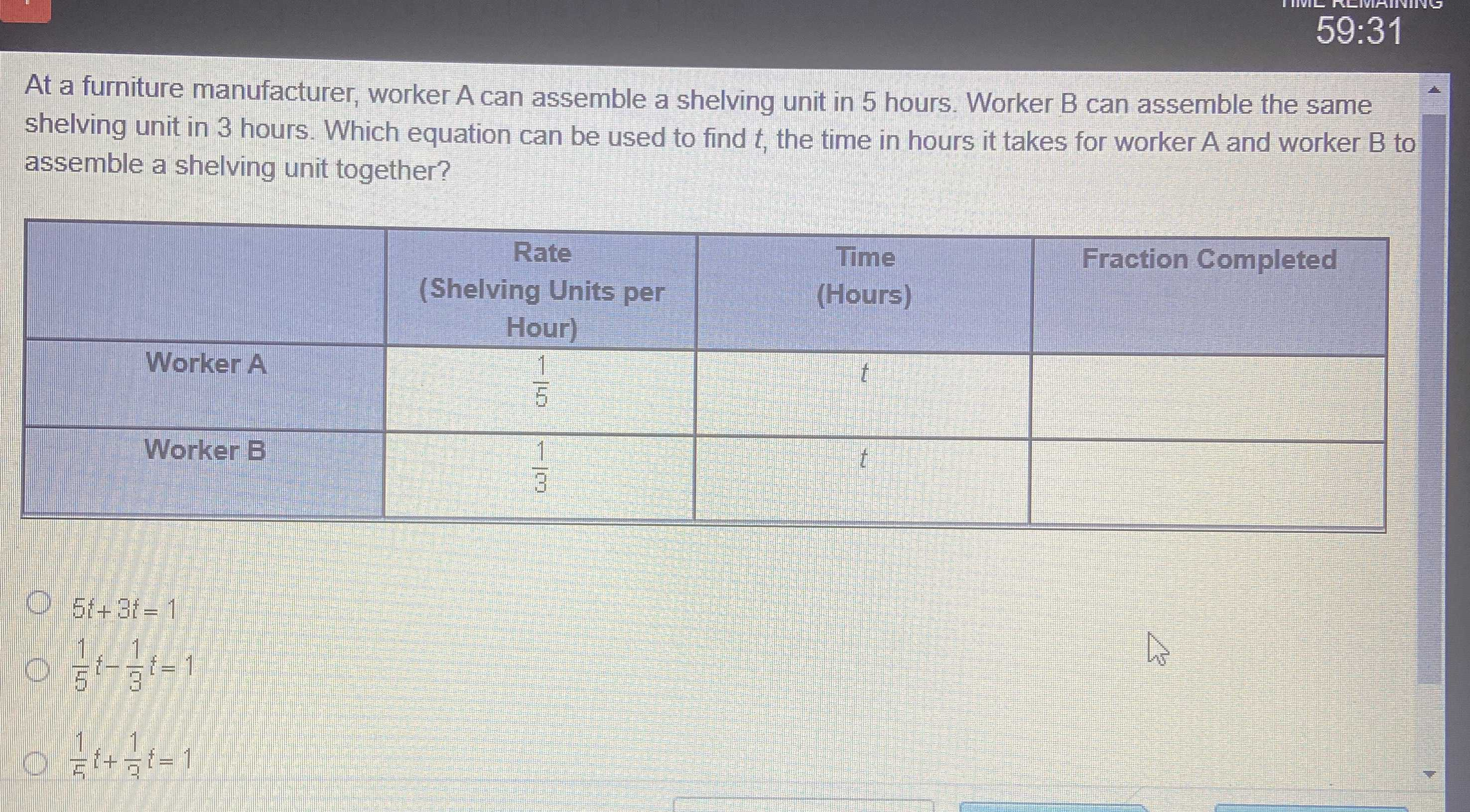### Still have math questions?

Algebra
QuestionAt a furniture manufacturer, worker A can assemble a shelving unit in $$5$$ hours. Worker B can assemble the same shelving unit in $$3$$ hours. Which equation can be used to find $$t$$ , the time in hours it takes for worker A and worker B to assemble a shelving unit together?

$$5 t + 3 t = 1$$

$$\frac { 1 } { 5 } t - \frac { 1 } { 3 } t = 1$$

$$\frac { 1 } { 5 } t + \frac { 1 } { 3 } t = 1$$